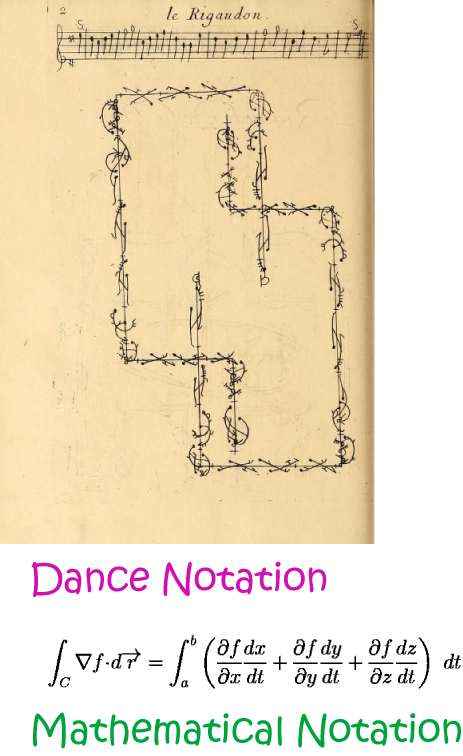# Definition of NotationNotation is a system of symbols that is used to communicate with other specialists in a field of study.

Notation is used in fields like music, mathematics and dance. The pictures show some examples of the use of notation in mathematics to make a statement precise, and notation in dance to describe the steps of a dance and how they fit with music.

For example, in mathematics we use the notation $\sqrt{4}$ to stand for the positive number that, when multiplied by itself, gives the answer $4$.

### Description

The aim of this dictionary is to provide definitions to common mathematical terms. Students learn a new math skill every week at school, sometimes just before they start a new skill, if they want to look at what a specific term means, this is where this dictionary will become handy and a go-to guide for a student.

### Audience

Year 1 to Year 12 students

### Learning Objectives

Learn common math terms starting with letter N

Author: Subject Coach
You must be logged in as Student to ask a Question.